Test: Linear Algebra - 2

# Test: Linear Algebra - 2

Test Description

## 20 Questions MCQ Test Topic-wise Tests & Solved Examples for IIT JAM Mathematics | Test: Linear Algebra - 2

Test: Linear Algebra - 2 for Mathematics 2022 is part of Topic-wise Tests & Solved Examples for IIT JAM Mathematics preparation. The Test: Linear Algebra - 2 questions and answers have been prepared according to the Mathematics exam syllabus.The Test: Linear Algebra - 2 MCQs are made for Mathematics 2022 Exam. Find important definitions, questions, notes, meanings, examples, exercises, MCQs and online tests for Test: Linear Algebra - 2 below.
Solutions of Test: Linear Algebra - 2 questions in English are available as part of our Topic-wise Tests & Solved Examples for IIT JAM Mathematics for Mathematics & Test: Linear Algebra - 2 solutions in Hindi for Topic-wise Tests & Solved Examples for IIT JAM Mathematics course. Download more important topics, notes, lectures and mock test series for Mathematics Exam by signing up for free. Attempt Test: Linear Algebra - 2 | 20 questions in 60 minutes | Mock test for Mathematics preparation | Free important questions MCQ to study Topic-wise Tests & Solved Examples for IIT JAM Mathematics for Mathematics Exam | Download free PDF with solutions
 1 Crore+ students have signed up on EduRev. Have you?
Test: Linear Algebra - 2 - Question 1

### If {v1, v2, v3} is a linearly independent set of vectors in a vector space over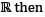which one of the following sets is also linearly independent?

Test: Linear Algebra - 2 - Question 2

### Consdierthe subspace W = {(x1, x2,.... ,x10)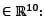xn = xn-1 + xn - 2 for 3 < n < 10) of the vector space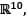the dimension of W is

Test: Linear Algebra - 2 - Question 3

### Which one of the following is a subspace of the vector space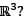Test: Linear Algebra - 2 - Question 4

If A and B are 3 × 3 real matrices such that rank (AB) = 1, then rank (BA) cannot be

Detailed Solution for Test: Linear Algebra - 2 - Question 4

Here A & B a re 3 × 2 real matrices such that rank (AB) = 1
So, |AB| = 0 ⇒ |A| |B| = 0 (∴ |AB| = |A| |B|)
⇒ either |A| or |B| should be zero
So, |BA| = |B||A| = 0
⇒ BA is singular
Hence rank (BA) cannot be 3. (Because BA is 3 × 3 matrix)

Test: Linear Algebra - 2 - Question 5

Which of the following sets of functions from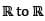is a vector space over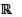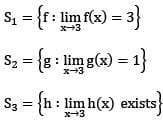Detailed Solution for Test: Linear Algebra - 2 - Question 5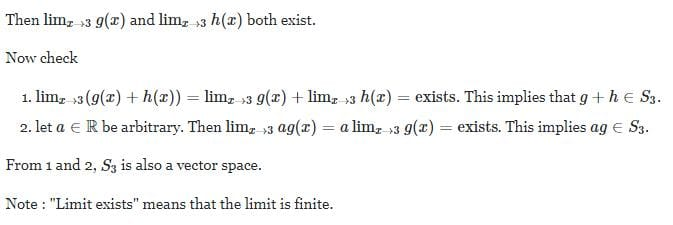Test: Linear Algebra - 2 - Question 6

Let T : R3 → R3 be the linear transformation define by T(x, y, z) = (x + y, + z, z + x) for all (x, y, z) ∈ 3. Then

Detailed Solution for Test: Linear Algebra - 2 - Question 6

Let T : R2 → R3 be the L.T. defined as
T (x, y, z) = (x + y, y + z, z + x) ∀ (x, y, z) ∈ R3
N (T ) = {( x, y, z)|T ( x, y, z) = 0}
= {(x, y, z)|x + y = 0,y + z = 0, z + x = 0}
= {(0, 0, 0)| x, y, z ∈R}
⇒ dim N (T) = 0
Rank (N) + Nullity T = dim R3 = 3
Rank (T) + 0 = 3 ⇒ Rank of T = 3

Test: Linear Algebra - 2 - Question 7

If the matrix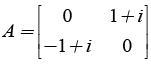the A

is

Detailed Solution for Test: Linear Algebra - 2 - Question 7

Here,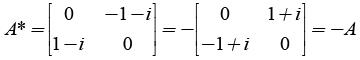Test: Linear Algebra - 2 - Question 8

Let V be a 3 dimensional vector space over the field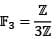of 3 elements. The number of distinct 1 dimensional subspaces of V is

Test: Linear Algebra - 2 - Question 9

Let M = {(a1,a2,a3): ai ∈ (1,2,3,4); a1 + a2 + a3 = 6} then the number of elements in M is

Test: Linear Algebra - 2 - Question 10

The dimension of the vector space of all symmetric matrices A = (aij) of order n x n (n > 2) with real entries, aii = 0 and trace zero is

Detailed Solution for Test: Linear Algebra - 2 - Question 10

The dimension of the space of n×n symmetric matrices with diagonal equal to zero is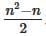. Now, in your case the diagonal is not zero but the sum of its elements is zero, that means that you have n−1 elements which can vary. SO you get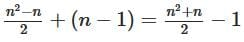as expected.

Test: Linear Algebra - 2 - Question 11

Let n be a positive integer and let Hn be the space of all n x n matrices A = (aij) with entries in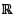satisfying aij = ars whenever i + j = r + s (i, j, r, s = 1, 2,..., n) then the dimention of Hn, as a vector space over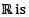Test: Linear Algebra - 2 - Question 12

The dimension of the vector space of all symmetric matrices of order n x n (n > 2) with real entries and trace equal to zero is

Test: Linear Algebra - 2 - Question 13

Let {X,Y,Z) be a basis of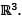Consider the following statements P and Q
P : {X + Y,Y + Z, X - Z) is a basis of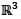Q : {X + Y + Z,X + 2Y - Z, X - 3Z} is a basis ofWhich of the above statements hold true?

Test: Linear Algebra - 2 - Question 14

Let V denote the vector space C5[a, b] over R and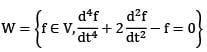Test: Linear Algebra - 2 - Question 15

Let M be the space of all 4 x 3 matrices with entries in the finite field of three elements. Then the number of matrices of Rank three In M is

Test: Linear Algebra - 2 - Question 16

Consider the subspace W = {[aij] : aij = 0, if i is even) of all 10 x 10 matrices(real) then the dimension of W is

Test: Linear Algebra - 2 - Question 17

The dimension of the vector space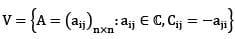over the field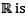Test: Linear Algebra - 2 - Question 18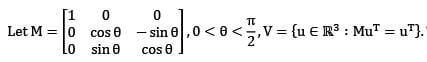Then the dim V is

Test: Linear Algebra - 2 - Question 19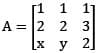and let V = {(x, y, z)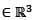: |A| = 0}.Then the dimension of V equals

Test: Linear Algebra - 2 - Question 20

A basis of V = {(x, y, z, w)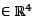: x + y - z = 0, y + z + w = 0, 2x + y - 3z = 0)

Detailed Solution for Test: Linear Algebra - 2 - Question 20

The system of 3 equations in 4 unknowns is

1x + 1y +(-1)z +0w =0……..(1)

0x + 1y + 1z + 1w = 0……….(2)

2x + 1y +(-3)z +1w = 0……..(3). The row operation (3) - 2×(1) reduces (3) to

0x +(-1)y+(-1)z+(-1)w =0…….(4), which is just (-1)× (3). Hence the given system is equivalent to the pair of equations:

x = -y+z and y= -z -w. This pair is equivalent to x=(z+w)+z = 2z+w and y = -z -w, where z and w are completely arbitrary. Thus

[x,y,z,w] =[2z+w,-z-w,z,w] =

z[2,-1,1,0] + w[1,-1,0,1].

We had already observed that the rank of the echelon matrix equivalent to the given coefficient matrix is 2, and hence its nullity = 4–2 = 2, which is the same as the dimension of the solution space V. Thus a basis of V may be taken as

B={[2,-1,1,0], [1,-1,0,1]}.

## Topic-wise Tests & Solved Examples for IIT JAM Mathematics

27 docs|150 tests
 Use Code STAYHOME200 and get INR 200 additional OFF Use Coupon Code
Information about Test: Linear Algebra - 2 Page
In this test you can find the Exam questions for Test: Linear Algebra - 2 solved & explained in the simplest way possible. Besides giving Questions and answers for Test: Linear Algebra - 2, EduRev gives you an ample number of Online tests for practice

## Topic-wise Tests & Solved Examples for IIT JAM Mathematics

27 docs|150 tests